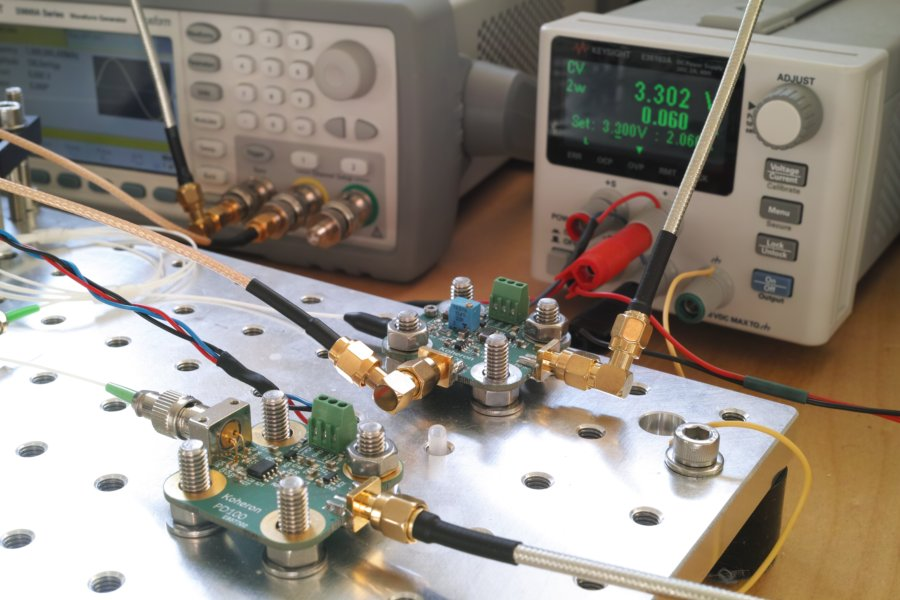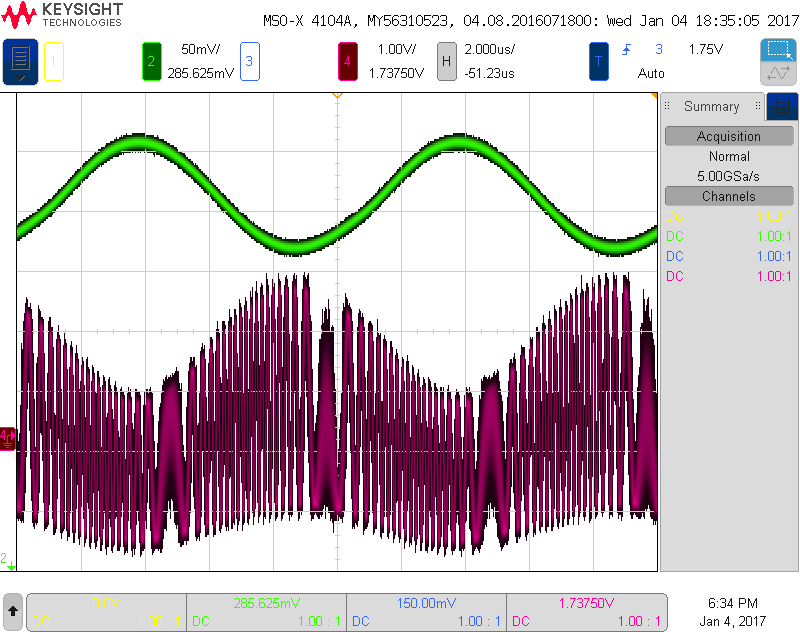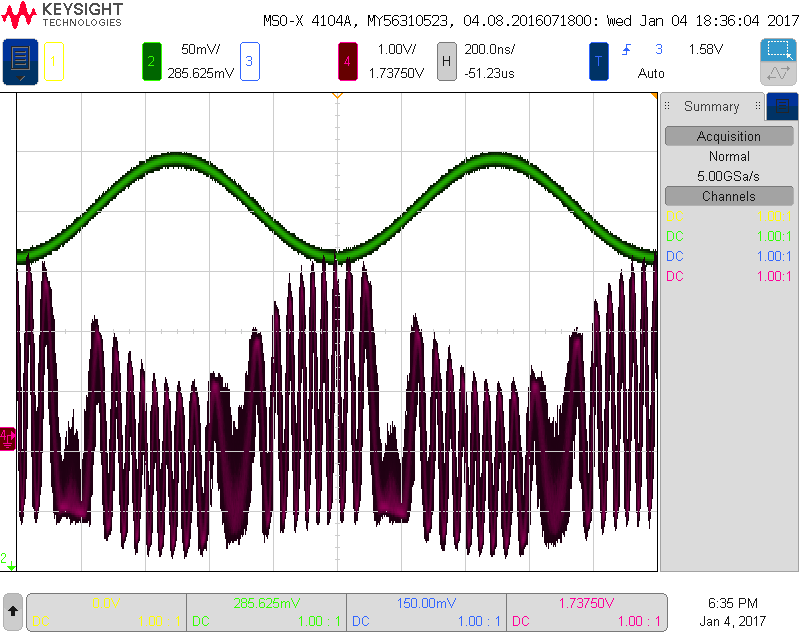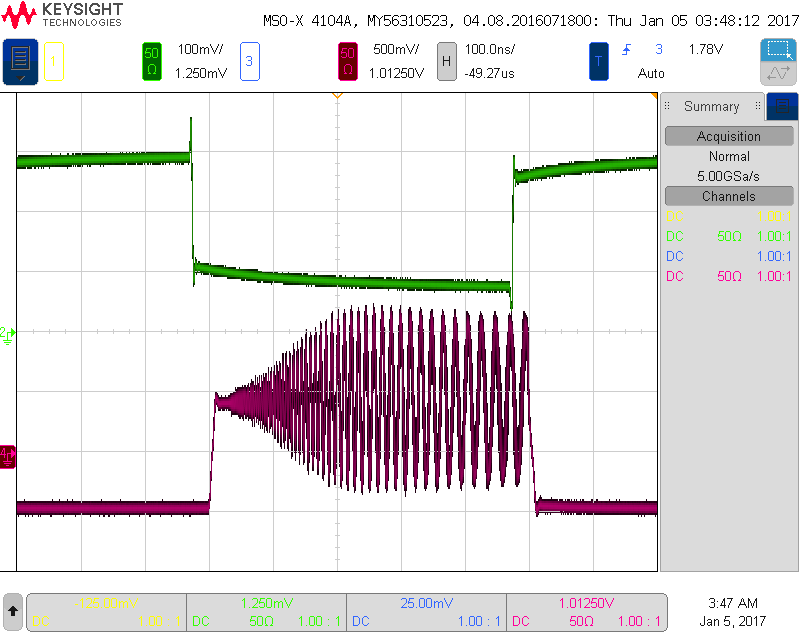# Amplitude and frequency modulation of a DFB laser

2017-01-05

In this post, we explore the effect of current modulation on the amplitude and frequency of the Koheron LD100 laser.The functional diagram of the LD100 laser is shown below. The current driver makes sure the laser operates at a stable DC current. The modulation input is AC-coupled with a 50 Ω resistor in series with a 1 µF capacitor. This corresponds to a cutoff frequency $1/2\pi RC$ = 3.2 kHz. Because of the 50 Ω termination, a 1 V modulation results in 20 mA modulation of the laser current.

## Experimental setup

We modulate the laser with a Keysight 33600A waveform generator. Amplitude modulation is observed with the photodetection integrated in the LD100 laser. Laser frequency modulation is observed with a Koheron PD100 photodetector at the output of a Mach-Zehnder interferometer with a 1 m fiber delay.The field at the interferometer output is $e_o(t) = e(t) + e(t-\tau)$, where $\tau$ is the 5 ns delay corresponding to the 1 m fiber and $e(t) = a(t) \exp(2i\pi f(t) t)$ is the laser field at time $t$. The photodetector output is proportional to $| e_o(t) | ^{2}$, averaged over the detector bandwidth:

$$u(t) = S A(t)^2 (1 + \cos(2 \pi \left[f(t) - f(t - \tau) \right] t + \tau f(t - \tau)) )$$,

where $S$ is a constant representing the detector sensitivity. We should thus observe interference fringes whose frequency is $f(t) - f(t - \tau)$.

## Small-signal modulation

We operate the laser with a DC current of 40 mA (IMON = 1.204 mV) and a sine wave modulation of 1 Vpp amplitude and 100 kHz frequency. The green curve in the figure below is the output of the LD100 integrated photodiode. It is inverted because of the negative transimpedance gain of -510 V/A. The red curve is the output of the PD100 photodetector.We observe that 19 interference fringes are scanned during half a period (5 µs). Since the interferometer delay is 5 ns, a fringe corresponds to a frequency shift of 200 MHz. The 1 Vpp modulation thus results in a frequency modulation of 3.8 GHz peak-peak. The minimum period of the interference fringes is about 175 ns. This means a maximum frequency slope of 1.14 GHz / µs.

The figure below was obtained with a sine wave modulation of 1 Vpp amplitude and 1 MHz frequency.We observe a minimum fringe period of 35 ns which corresponds to a maximum frequency slope of 5,7 GHz / µs.

Note that we cannot tell whether the laser frequency increases or decreases by only looking at the interference fringes. A solution is to shift the frequency of one arm of the interferometer with an acousto-optic modulator to observe the modulation around a carrier. However, we can say that the laser wavelength is proportional to the optical length of the laser cavity. As a result, the laser frequency should decrease with increasing current because of thermal expansion.

## Large-signal modulation

Finally, we modulate the laser with a 5 Vpp square wave of frequency 1 MHz.At the start of the pulse, the contrast of the fringes is limited by the 150 MHz bandwidth of the PD100 photodetector. The maximum contrast is at a fringe frequency of 70 MHz, which is in agreement with the peaking in the frequency response of the PD100 photodetector. The minimum observable fringe period is less than 1 ns. The frequency slope is thus higher than 40 GHz / µs at the beginning of the pulse.